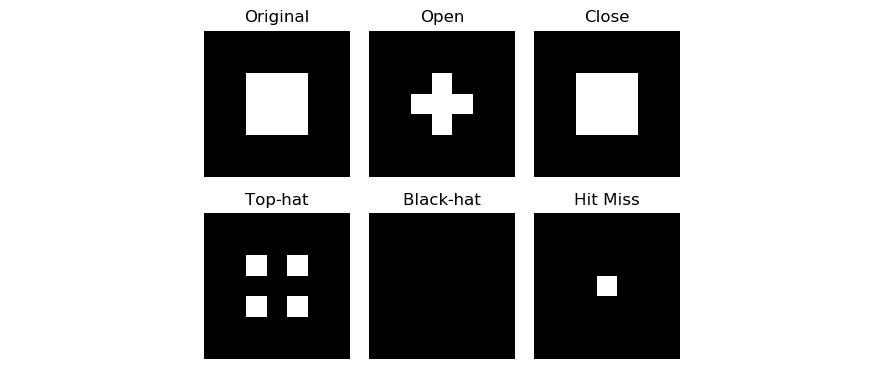• 计算和可视化直方图
• 可视化图像轮廓
• 将形态学变换应用于图像

# 计算和可视化直方图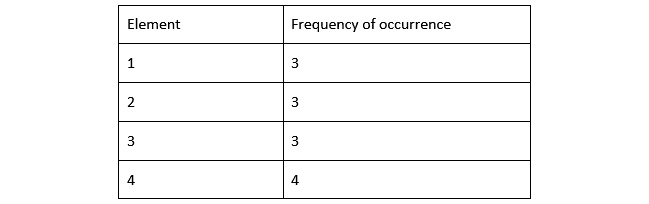import numpy as np
import matplotlib.pyplot as plt
a = np.array([1, 2, 1, 3, 4, 1, 2, 3, 4, 4, 2, 3, 4])
hist, bins = np.histogram(a)
print(hist)
print(bins)
plt.hist(a)
plt.show()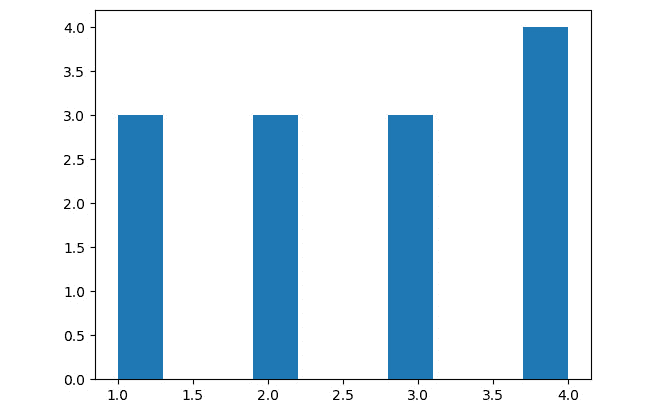import numpy as np
import matplotlib.pyplot as plt
import cv2
plt.subplot(1, 2, 1)
plt.imshow(img, cmap='gray')
plt.axis('off')
plt.title('Original Image')
plt.subplot(1, 2, 2)
hist, bins = np.histogram(img.ravel(),
bins=256,
range=(0, 255))
plt.bar(bins[:-1], hist)
plt.title('Histogram')
plt.show()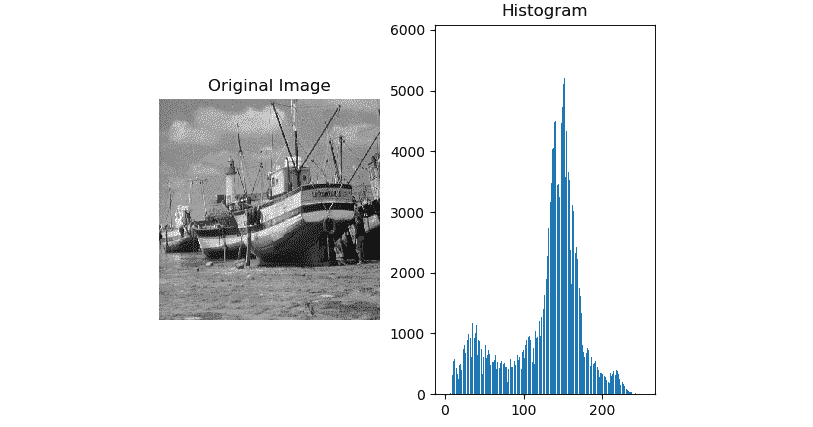plt.hist(img.ravel(), bins=256, range=(0, 255))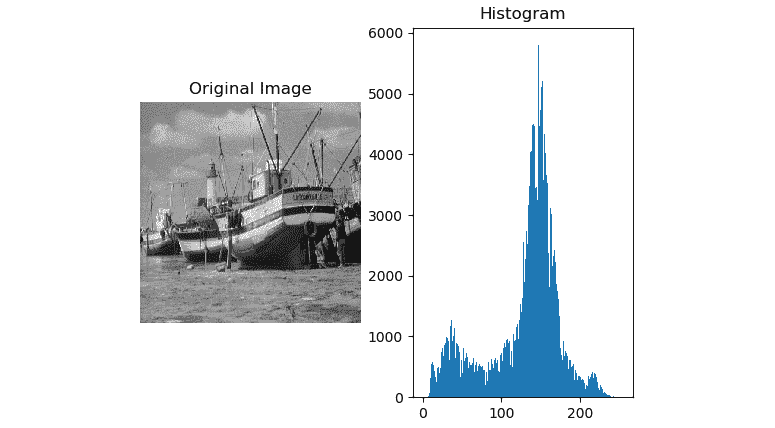import numpy as np
import matplotlib.pyplot as plt
import cv2
b = img[:, :, 0]
g = img[:, :, 1]
r = img[:, :, 2]
plt.subplot(2, 2, 1)
plt.imshow(cv2.cvtColor(img, cv2.COLOR_BGR2RGB),
cmap='gray')
plt.axis('off')
plt.title('Original Image')
plt.subplot(2, 2, 2)
plt.hist(r.ravel(), bins=256, range=(0, 255), color='r')
plt.title('Red Histogram')
plt.subplot(2, 2, 3)
plt.hist(g.ravel(), bins=256, range=(0, 255), color='g')
plt.title('Green Histogram')
plt.subplot(2, 2, 4)
plt.hist(b.ravel(), bins=256, range=(0, 255), color='b')
plt.title('Blue Histogram')
plt.show()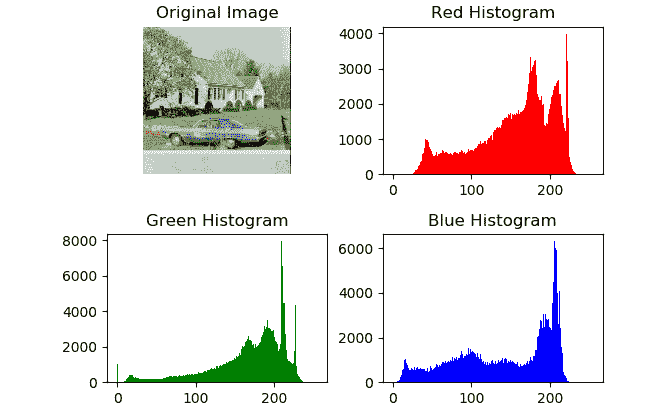OpenCV 提供cv2.calcHist()函数，以分别计算和可视化彩色图像的直方图通道。 cv2.calcHist()函数接受图像数组，掩码，通道索引，范围和大小作为参数，以为彩色图像的单个通道计算直方图。 以下示例通过分别计算和可视化每个颜色通道的直方图来演示：

import cv2
from matplotlib import pyplot as plt
input=cv2.cvtColor(img, cv2.COLOR_RGB2BGR)
histr_RED = cv2.calcHist([input], , None, , [0, 255])
histr_GREEN = cv2.calcHist([input], , None, , [0, 255])
histr_BLUE = cv2.calcHist([input], , None, , [0, 255])


plt.subplot(221)
plt.imshow(input)
plt.title('Original Image')
plt.axis('off')
plt.subplot(222)
plt.plot(histr_RED, color='r'),
plt.title('Red')
plt.xlim([0, 255])
plt.yticks([])
plt.subplot(223)
plt.plot(histr_GREEN, color='g')
plt.title('Green')
plt.xlim([0, 255])
plt.yticks([])
plt.subplot(224)
plt.plot(histr_BLUE, color='b')
plt.title('Blue')
plt.xlim([0, 255])
plt.yticks([])
plt.show()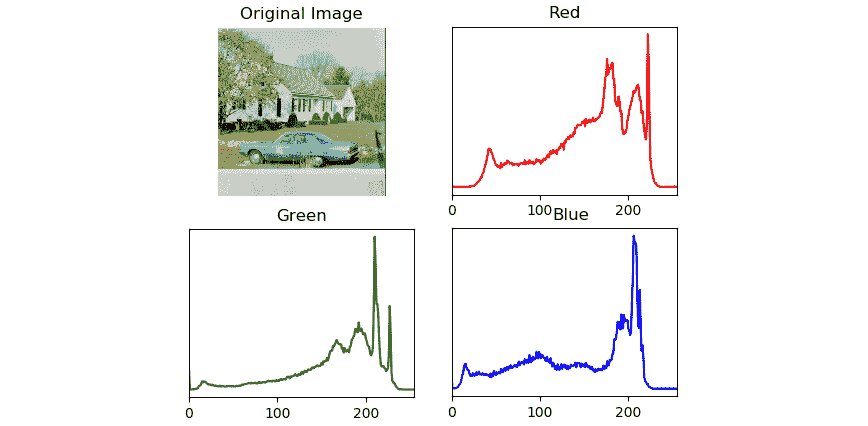## 直方图均衡

1. 在下面的代码中，我们演示了如何均衡 RGB 图像各个通道的直方图，然后再次合并它们以获得对比度增强的输出彩色图像：

py import cv2

py import matplotlib.pyplot as plt

py img = cv2.imread('/home/pi/book/dataset/4.2.03.tiff', 1)

py img = cv2.cvtColor(img, cv2.COLOR_BGR2RGB)

py R, G, B = cv2.split(img)

2. 让我们分别均衡图像的色彩通道，然后合并均衡通道以创建均衡图像：

py output1_R = cv2.equalizeHist(R)

py output1_G = cv2.equalizeHist(G)

py output1_B = cv2.equalizeHist(B)

py output1 = cv2.merge((output1_R,

py output1_G, output1_B))

3. 现在，让我们使用 CLAHE 方法来均衡颜色通道，然后将它们合并以获得带有 CLAHE 的均衡图像：

py clahe = cv2.createCLAHE(clipLimit=2.0,

py tileGridSize=(8, 8))

py output2_R = clahe.apply(R)

py output2_G = clahe.apply(G)

py output2_B = clahe.apply(B)

py output2 = cv2.merge((output2_R, output2_G,

py output2_B))

py output = [img, output1, output2]

py titles = ['Original Image',

py 'Adjusted Histogram', 'CLAHE']

py for i in range(3):

py plt.subplot(1, 3, i+1)

py plt.imshow(output[i])

py plt.title(titles[i])

py plt.axis('off')

py plt.show()

两种方法的输出如下。 第一个是原始图像，第二个是直方图调整后的图像，第三个图像是使用 CLAHE 生成的直方图均衡图像：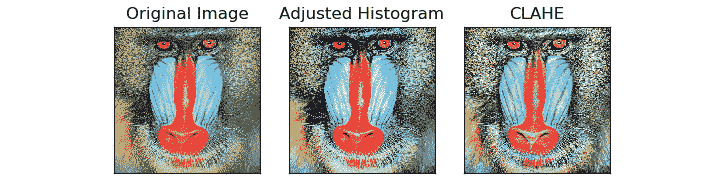# 可视化图像轮廓

cv2.findContours()函数用于计算图像中的轮廓。 该函数接受图像数组，轮廓检索模式以及将轮廓逼近作为参数的方法。 然后，它返回图像中计算机轮廓的列表。 轮廓检索模式可以是以下任意一种：

• CV_RETR_CCOMP
• CV_RETR_TREE
• CV_RETR_EXTERNAL
• CV_RETR_LIST

• CV_CHAIN_APPROX_TC89_L1
• CV_CHAIN_APPROX_TC89_KCOS
• CV_CHAIN_APPROX_NONE
• CV_CHAIN_APPROX_SIMPLE

import cv2
import matplotlib.pyplot as plt
img = cv2.cvtColor(img, cv2.COLOR_BGR2RGB)
gray = cv2.cvtColor(img, cv2.COLOR_BGR2GRAY)
ret, thresh = cv2.threshold(gray, 75, 255, 0)
contours, hierarchy = cv2.findContours(thresh, cv2.RETR_TREE,
cv2.CHAIN_APPROX_SIMPLE)
cv2.drawContours(img, contours, -1, (0, 0, 255), 2)
original = cv2.cvtColor(original, cv2.COLOR_BGR2RGB)
output = [original, img]
titles = ['Original', 'Contours']
for i in range(2):
plt.subplot(1, 2, i+1)
plt.imshow(output[i])
plt.title(titles[i])
plt.axis('off')
plt.show()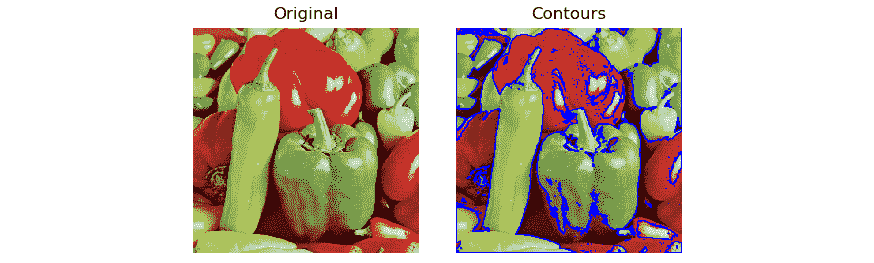# 对图像执行形态转换

import numpy as np
import cv2
from matplotlib import pyplot as plt
Let us create a sample image,img = np.array([[0, 0, 0, 0, 0, 0, 0],
[0, 0, 0, 0, 0, 0, 0],
[0, 0, 255, 255, 255, 0, 0],
[0, 0, 255, 255, 255, 0, 0],
[0, 0, 255, 255, 255, 0, 0],
[0, 0, 0, 0, 0, 0, 0],
[0, 0, 0, 0, 0, 0, 0]], dtype=np.uint8)


kernel = np.ones((3, 3), np.uint8)
erosion = cv2.erode(img, kernel, iterations = 1)
dilation = cv2.dilate(img, kernel, iterations = 1)
kernel)
titles=['Original', 'Erosion',


for i in range(4):
plt.subplot(2, 2, i+1)
plt.imshow(output[i], cmap='gray')
plt.title(titles[i])
plt.axis('off')
plt.show()1. 以交互方式打开 Python 3 并运行以下语句：

py

import cv2


py

k = cv2.getStructuringElement(cv2.MORPH_RECT, (5, 5)) 

py

k 

输出如下：

py array([[1, 1, 1, 1, 1],

py [1, 1, 1, 1, 1],

py [1, 1, 1, 1, 1],

py [1, 1, 1, 1, 1],

py [1, 1, 1, 1, 1]], dtype=uint8)

2. 让我们看一个椭圆的结构元素：

py

k = cv2.getStructuringElement(cv2.MORPH_ELLIPSE,(5, 5)) 

py

k 

输出如下：

py array([[0, 0, 1, 0, 0],

py [1, 1, 1, 1, 1],

py [1, 1, 1, 1, 1],

py [1, 1, 1, 1, 1],

py [0, 0, 1, 0, 0]], dtype=uint8)

3. 让我们看一个跨结构元素：

py

k = cv2.getStructuringElement(cv2.MORPH_CROSS,(5, 5)) 

py

k 

输出如下：

py array([[0, 0, 1, 0, 0],

py [0, 0, 1, 0, 0],

py [1, 1, 1, 1, 1],

py [0, 0, 1, 0, 0],

py [0, 0, 1, 0, 0]], dtype=uint8)

让我们通过使用自定义3x3交叉核查看其余的形态学操作。

4. 让我们导入所有必需的库：

py import numpy as np

py import cv2

py from matplotlib import pyplot as plt

5. 以下几行创建一个示例二进制图像：

py img = np.array([[0, 0, 0, 0, 0, 0, 0],

py [0, 0, 0, 0, 0, 0, 0],

py [0, 0, 255, 255, 255, 0, 0],

py [0, 0, 255, 255, 255, 0, 0],

py [0, 0, 255, 255, 255, 0, 0],

py [0, 0, 0, 0, 0, 0, 0],

py [0, 0, 0, 0, 0, 0, 0]], dtype=np.uint8)

6. 现在让我们为结构元素创建矩阵：

py kernel = cv2.getStructuringElement(cv2.MORPH_CROSS, (3, 3))

7. 现在，我们将数学形态学操作应用于样本二进制图像：

py open = cv2.morphologyEx(img,

py cv2.MORPH_OPEN,

py kernel)

py close = cv2.morphologyEx(img,

py cv2.MORPH_CLOSE,

py kernel)

py tophat = cv2.morphologyEx(img,

py cv2.MORPH_TOPHAT,

py kernel)

py blackhat = cv2.morphologyEx(img,

py cv2.MORPH_BLACKHAT,

py kernel)

[

py hitmiss = cv2.morphologyEx(img,

py cv2.MORPH_HITMISS,

py kernel)

8. 现在让我们可视化输入和输出：

py titles=['Original', 'Open',

py 'Close', 'Top hat',

py 'Black hat', 'Hit Miss']

py output=[img, open, close,

py tophat, blackhat,

py hitmiss]

py for i in range(6):

py plt.subplot(2, 3, i+1)

py plt.imshow(output[i], cmap='gray')

py plt.title(titles[i])

py plt.axis('off')

py plt.show()

前面的代码的输出如下：